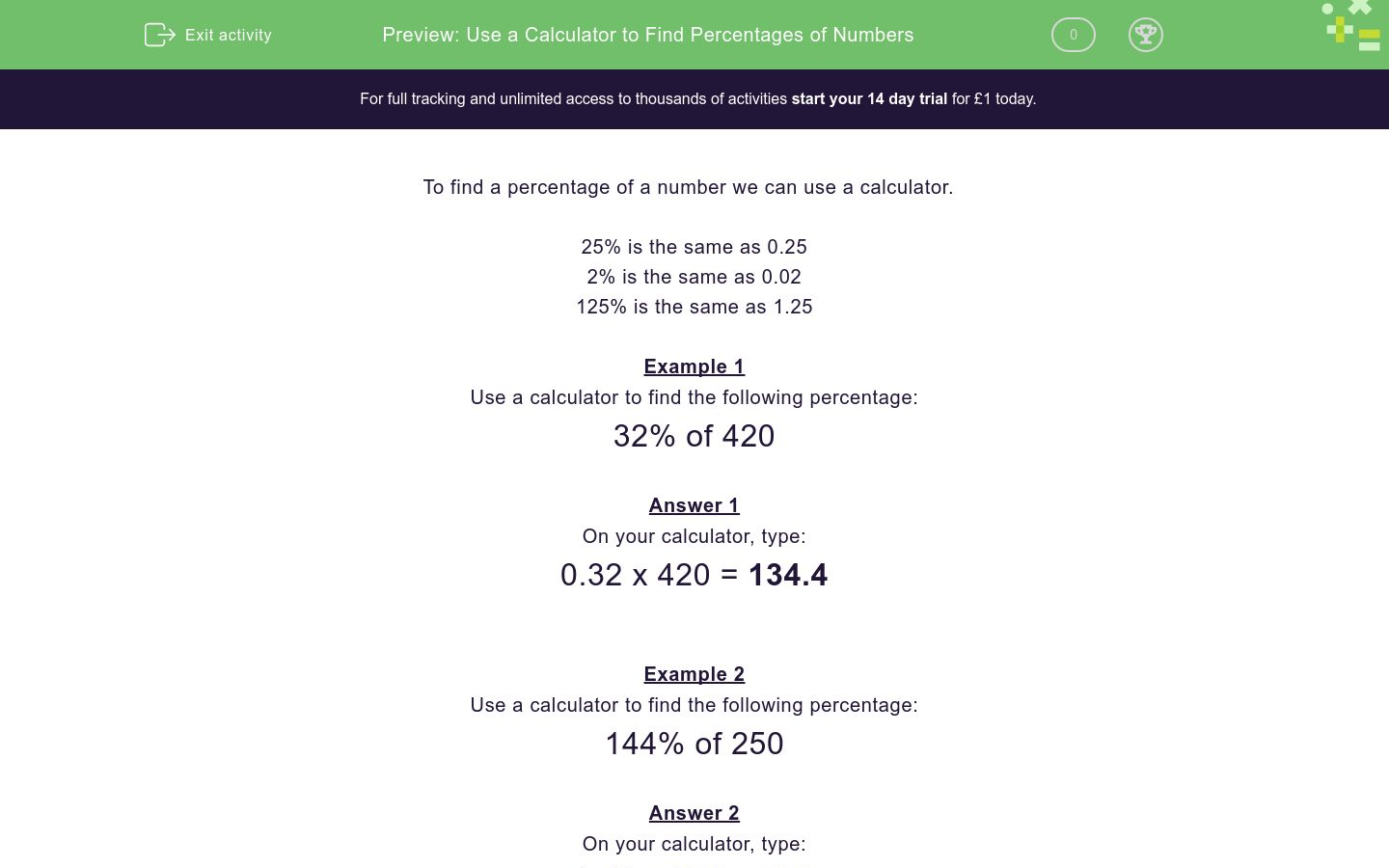# Use a Calculator to Find Percentages of Numbers

In this worksheet, students use a calculator to find percentages of numbers.Key stage:  KS 3

Curriculum topic:   Number

Curriculum subtopic:   Use Calculators/Technology for Accuracy

Difficulty level:### QUESTION 1 of 10

To find a percentage of a number we can use a calculator.

25% is the same as 0.25

2% is the same as 0.02

125% is the same as 1.25

Example 1

Use a calculator to find the following percentage:

32% of 420

0.32 x 420 = 134.4

Example 2

Use a calculator to find the following percentage:

144% of 250

1.44 x 250 = 360

Use a calculator to find the following percentage:

28% of 91

Use a calculator to find the following percentage:

4% of 75

Use a calculator to find the following percentage:

4% of 130

Use a calculator to find the following percentage:

129% of 139

Use a calculator to find the following percentage:

13% of 104

Use a calculator to find the following percentage:

149% of 242

Use a calculator to find the following percentage:

87% of 77

Use a calculator to find the following percentage:

58% of 155

Use a calculator to find the following percentage:

26% of 216

Use a calculator to find the following percentage:

128% of 94

• Question 1

Use a calculator to find the following percentage:

28% of 91

25.48
EDDIE SAYS
28% is a multiplier of 0.28 0.28 × 91 = 25.48
• Question 2

Use a calculator to find the following percentage:

4% of 75

3
EDDIE SAYS
4% is a multiplier of 0.04 0.04 × 75 = 3
• Question 3

Use a calculator to find the following percentage:

4% of 130

5.2
EDDIE SAYS
4% is a multiplier of 0.04 0.04 × 130 = 5.2
• Question 4

Use a calculator to find the following percentage:

129% of 139

179.31
EDDIE SAYS
129% is a multiplier of 1.29 1.29 × 139 = 179.31
• Question 5

Use a calculator to find the following percentage:

13% of 104

13.52
EDDIE SAYS
13% is a multiplier of 0.13 0.13 × 104 = 13.52
• Question 6

Use a calculator to find the following percentage:

149% of 242

360.58
EDDIE SAYS
149% is a multiplier of 1.49 1.49 × 242 = 360.58
• Question 7

Use a calculator to find the following percentage:

87% of 77

66.99
EDDIE SAYS
87% is a multiplier of 0.87 0.87 × 77 = 66.99
• Question 8

Use a calculator to find the following percentage:

58% of 155

89.9
EDDIE SAYS
58% is a multiplier of 0.58 0.58 × 155 = 89.9
• Question 9

Use a calculator to find the following percentage:

26% of 216

56.16
EDDIE SAYS
26% is a multiplier of 0.26 0.26 × 216 = 56.16
• Question 10

Use a calculator to find the following percentage:

128% of 94

120.32
EDDIE SAYS
128% is a multiplier of 1.28 1.28 × 94 = 120.32
---- OR ----